Courses

# Test: Syntax Directed Translation- 1

## 20 Questions MCQ Test RRB JE for Computer Science Engineering | Test: Syntax Directed Translation- 1

Description
This mock test of Test: Syntax Directed Translation- 1 for Computer Science Engineering (CSE) helps you for every Computer Science Engineering (CSE) entrance exam. This contains 20 Multiple Choice Questions for Computer Science Engineering (CSE) Test: Syntax Directed Translation- 1 (mcq) to study with solutions a complete question bank. The solved questions answers in this Test: Syntax Directed Translation- 1 quiz give you a good mix of easy questions and tough questions. Computer Science Engineering (CSE) students definitely take this Test: Syntax Directed Translation- 1 exercise for a better result in the exam. You can find other Test: Syntax Directed Translation- 1 extra questions, long questions & short questions for Computer Science Engineering (CSE) on EduRev as well by searching above.
QUESTION: 1

### To evaluate an expression without any embedded function calls

Solution:

► Expression without any calls in it ⇒ 1+2*3-4

► Expression with embedded calls ⇒ 1 + fun1(a,b,c) *fun2(3.4,58) - fun3(x,yz);

First we can convert Infix to Postfix using single stack (Using it as operator stack)

Then we can evaluate that expression using Single stack.

*Answer can only contain numeric values
QUESTION: 2

### Consider the expression tree shown. Each leaf represents a numerical value, which can either be 0 or 1. Over all possible choices of the values at the leaves, the maximum possible value of the expression represented by the tree is ___.Solution:

at left  leafs
+   -----> (1,1)=2         intermediate + ------->  2-(-1)=3    -  -------->(0,1)=-1
at right leafs
-  minus ------->(1,0)=1       intermediate   +    ------> 1+2=3 +     ------------->(1,1)=2
at root   + -------> 3+3=6

QUESTION: 3

### Which one of the following grammars is free from left recursion?

Solution: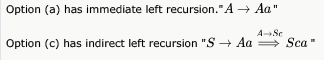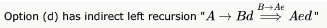Option (b) is free from left recursion. No direct left recursion. No indirect left recursion.

QUESTION: 4

The number of possible min-heaps containing each value from {1, 2, 3, 4, 5, 6, 7} exactly once is _______.

Solution:

Set minimum element as root (i.e 1), now 6 are remaining and left subtree will have 3 elements, each left subtree combination can be permuted in 2! ways.

Total ways to design min-heap with 7-elements =*2! * 2! = 20*2*2 = 80

Alternative approach – Total number of min or max heap tree with 1 to N elements are using recurrence relation:

• T(N) = (N-1)Ck * T(k) * T(N-k-1), were k = number of nodes on left subtree
•  T(1) = 1 T(2) = 1 T(3) = 2 T(4) = 3C2 * T(2) * T(1) = 3 T(5) = 4C3 * T(3) * T(1) = 8 T(6) = 5C3 * T(3) * T(2) = 20 T(7) = 5C3 * T(3) * T(3) = 80

QUESTION: 5

Which of the following features cannot be captured by context-free grammars?

Solution:

Since CFG's are used to show syntactic rules while designing compiler, and syntactic rules don't check for meaningful things such as if a variable has been declared before its use or not. Such things are meant to be handled by Semantic Analysis phase (requires power of a context sensitive grammar).
For D, a regular expression does not restrict the string length. Languages have restriction for variable name length for storing purpose like in symbol table.
For A, if then else is inherently ambiguous. But CFG can represent inherently ambiguous languages just that there are more than one parse trees possible for some strings.

QUESTION: 6

Consider a grammar with the following productions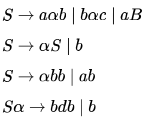The above grammar is:

Solution:

S∝ ->

This violates the conditions of context-free and hence the grammar becomes context-sensitive.

QUESTION: 7

The grammar whose productions are

-> if id then <stmt>

-> if id then <stmt> else <stmt>

-> id := id

is ambiguous because

Solution:

if a then if b then c: = d else c:= f  has two parse trees as follows:

1. if a then (if b then c:= d) else c:= f 2. and if a then (if b then c:=d else c:= f)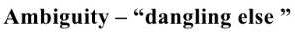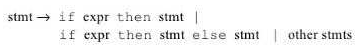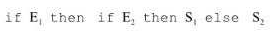QUESTION: 8

In the following grammar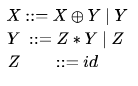Which of the following is true?

Solution:

It will be A. For multiple '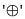', the derivation is possible only via ' ' which is on left side of '' in the production. Hence it is left associative.

For multiple '* ', the derivation is possible only via '* ' which is on the right side of ' ' in the production. Hence it is right associative.

If both left and right derivations were possible, the grammar would have been ambiguous and we couldn't have given associativity.

QUESTION: 9

A grammar that is both left and right recursive for a non-terminal, is:

Solution:

Let grammar is like this :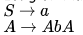This grammar is left as well as right recursive but still unambiguous.. A is useless production but still part of grammar..
A grammar having both left as well as right recursion may or may not be ambiguous ..

Let's take a grammar say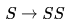Now, according to the link https://en.wikipedia.org/wiki/Formal_grammar

For a grammar G, if we have L(G) then all the strings/sentences in this language can be produced after some finite number of steps .

But, for the grammarCan we produce any string after a finite number of steps ? NO, and hence language of this grammar is empty set {} .

Hence, If a grammar is having both left and right recursion, then grammar may or may not be ambiguous .

QUESTION: 10

Given the following expression grammar: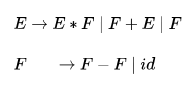Which of the following is true?

Solution:

Operator which is at lower level in the grammar is termed to have higher precedence.

QUESTION: 11

Which of the following statements is false?

Solution:

(A) We can not have different Left Most Derivation and Right Most Derivation parse trees BUT we can certainly have different LMD and RMD for a given string.(LMD and RMD here refers to the order of various productions used for derivation which could be different.)

(D) is wrong w.r.t. question because IT IS TRUE that any LR(k) IS NEVER AMBIGUOUS and so an ambiguous can never be an LR(K) for any k, no matter how large k becomes.

(B) and (C) can not be the answer because LL(1) is TOP-DOWN parser and LALR is powerful than SLR. So both are TRUE.

QUESTION: 12

Consider the grammar shown below

S → i E t S S’ | a
S’ → e S | ε
E → b

In the predictive parse table, M, of this grammar, the entries M[S’ , e] and M[S’ , \$] respectively are

Solution: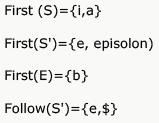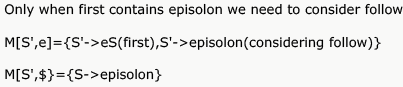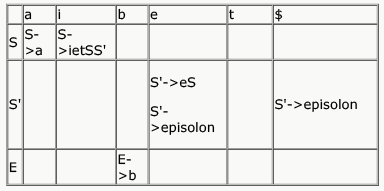QUESTION: 13

Consider the translation scheme shown below.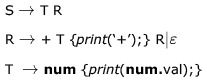Here num is a token that represents an integer and num.val represents the corresponding integer value. For an input string ‘9 + 5 + 2’, this translation scheme will print

Solution:

Let us make the parse tree for 9+5+2 in top down manner, left first derivation.

Steps:

1. Exapnd S-> TR
2. apply T-> Num...
3. apply R -> +T...
4. apply T->Num...
5. apply R-> +T..
6. apply T-> Num..
7. apply R-> epsilon

After printing through the print statement in the parse tree formed you will get the answer as 9 5 + 2 +.

QUESTION: 14

Consider the grammar with the following translation rules and E as the start symbol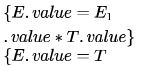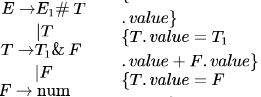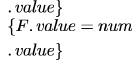Compute E.value for the root of the parse tree for the expression:2 # 3 & 5 # 6 & 4

Solution:

We can do it in a simple manner.

Here # is multiplication and & is addition by semantics rules given in the question and by observation of productions.

1. here &(+) is higher precedence than #(*). bcoz & is far from starting symbol.
2. and both &,# are left associative so we can solve the express in this way ((2*(3+5))*(6+4)) =160 so ans should be (C).
QUESTION: 15

Which of the following grammar rules violate the requirements of an operator grammar? P, Q, R are nonterminals, and r, s, t are terminals.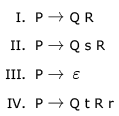Solution:

because operator grammer  does not contain  1)  nullable variable  2) 2 adjacent non-terminal on rhs of production

QUESTION: 16

Consider the following grammar G: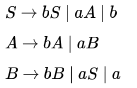Let Na(ω) and Nb(ω)  denote the number of a’s and b’s in a string ω respectively.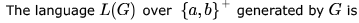Solution:

above CFG generate string b, aaa.. b will eliminate options A and D aaa eliminate options B.
C is answer i.e. number of a = 3k, k = 0,1,2....

QUESTION: 17

Consider the grammar: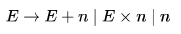For a sentence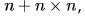, the handles in the right-sentential form of the reduction are:

Solution:

n+n*n

E+n*n

E*n

E

string in red indicates handle here

QUESTION: 18

Consider the following statements about the context free grammar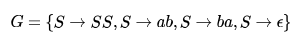I. G is ambiguous
II. G produces all strings with equal number of a's and b's
III. G can be accepted by a deterministic PDA

Which combination below expresses all the true statements about ?

Solution:

1.G is ambiguous. E.g. the string  has multiple derivation trees like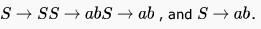II. False.  does not produce all strings with equal no. of `s and `s. ( cannot be generated).

III. True. The given grammar G generates the language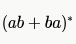, which is Regular and therefore also DCFL. So, a DPDA can be designed for G .

QUESTION: 19

Which one of the following grammars generates the language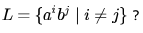Solution: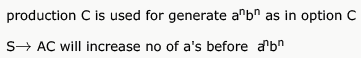as A will generate a or aa or aaa... i,e a+ ,  so S→ AC will generate a+anbn , i.e aibj i>jQUESTION: 20

Consider the grammar with non-terminals N= {S,C,S1} terminals T = {a,b,i,t,e} with S as the start symbol, and the following set of rules: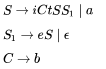The grammar is NOT LL(1) because:

Solution:

A  LL(1) grammar doesn’t give to multiple entries in a single cell of its parsing table. It has only single entry in a single cell, hence it should be unambiguous.

Option A is wrong. Grammar is not left recursive. For a grammar to be left recursive a production should be of form A->Ab, where A is a single Non-Terminal and b is any string of grammar symbols.

Option B is wrong. Because a right recursive grammar has nothing to do with LL(1).

Option D is wrong. Because the given grammar is clearly a Context Free Grammar. A grammar is CFG if it has productions of the form A->(V∪ T)* , where A is a single non-terminal and V is a set of Non-terminals and T is a set of Terminals.

Hence Option C should be the correct one. i.e. the grammar is ambiguous.

But let’s see how the grammar is ambiguous.

If the grammar is ambiguous then it should give multiple entry in a cell while making its parsing table. And Parse table is made with the aid of two functions : FIRST and FOLLOW.

A parsing table of a grammar will not have multiple entries in a cell( i.e. will be a LL(1) grammar) if and only if the following conditions hold for each production of the form A->α|β

1) FIRST(α)  ∩ FIRST(β) =   Φ

2) if FIRST(α) contains ‘ ε ‘ then FIRST(α) ∩ FOLLOW (A) =  Φ and vice-versa.

Now,

• For the production , S->iCtSS1|a, rule 1 is satisfied, because FIRST(iCtSS1) ∩ FIRST(a) = {i} ∩ {a} = Φ
• For the production, S1->eS|ε, rule 1 is satisfied, as FIRST(eS) ∩ FIRST(ε) = {e} ∩ {ε} = Φ   .  But here due to ‘ε’ in FIRST, we have to check for rule 2. FIRST(eS)  ∩ FOLLOW(S1) = {e} ∩ {e, \$} ≠ Φ .  Hence rule 2 fails in this production rule. Therefore there will be multiple entries in the parsing table, hence the grammar is ambiguous and not LL(1).